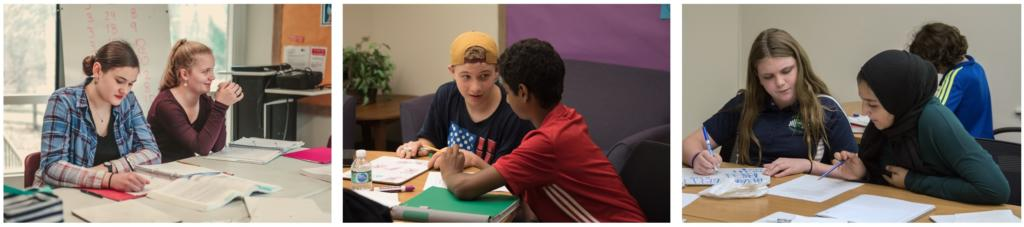# Math Roadblocks at Home? Try Math Lab at Compass

## Math Lab is one way to make sure the math work is getting done…

Math Lab is a tutoring center where students can go for weekly help on math homework! Math Lab is held on Wednesdays* at 2:00 pm. An experienced Compass math teacher provides on-the-spot help with math homework for any middle school math or high school math topic.

In Math Lab, students bring the math homework they are assigned- whether from a Compass math class, an online math program, or material taught at home. Sometimes just having a helper nearby or hearing a math explanation from someone else will make the concept “click.”

How does Math Lab work?

• Bring a current math assignment including any textbook, workbook, or worksheets
• Work independently until you have a question, reach a stumbling block, or need clarification on a concept or computation.
• An experienced Compass math instructor will be circling the room and continually checking in with students.
• The tutor will help students check answers, remind them of a technique, or demonstrate a different way to solve the problem.
• A partial list of topics that students can receive help on includes: number lines, integers, negative numbers, fractions, decimals, percentages, factoring, exponents, roots, order of operations, inequalities, coordinate plane, working with variables, solving equations, and word problems.

Register for Math Lab by 7 or 8- week quarters. Register for 2nd quarter Math Lab online starting October 6. Contact Compass to enroll in Math Lab for the remainder of first quarter. Math Lab is a fraction of the cost of a private math tutor. *Additional days and times will be added as the number of Math Lab students increases.